﻿ 一种基于最小距离和稀疏图正则约束的非负矩阵解混算法

一种基于最小距离和稀疏图正则约束的非负矩阵解混算法A Nonnegative Matrix Unmixing Algorithm Based on Minimum Distance and Sparse Graph Regularity

Abstract: Aiming at the problems of non-negative matrix unmixing model, such as easy to fall into the local minimum, being greatly affected by noise, and low unmixing accuracy in the case of “foreign matter having the same spectrum” end elements in mixed pixels, etc., in this paper, a non-negative matrix factorization algorithm (DLGNMF) based on minimum distance and regular constraints of sparse graphs is proposed. The algorithm combines spatial information with spectral information. In the objective function, the constraint of minimizing the distance of a single body is introduced as the constraint condition of the end-member. The sparsity constraint and graph regularization constraint are taken as the constraints of abundance, which ensure the global sparsity and local similarity of the end-member distribution in the hyperspectral image, solve the problems of non-negative matrix model and improve the accuracy of solution unmixing.

1. 引言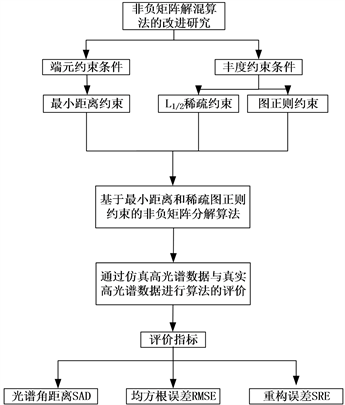Figure 1. Algorithm flow chart

2. 理论介绍

2.1. 线性混合的高光谱非负矩阵解混模型

$X=AS+n$ (1)

$\mathrm{min}f\left(A,S\right)=\frac{1}{2}{‖X-AS‖}_{F}^{2}$ (2)

$A←A\frac{X{S}^{\text{T}}}{AS{S}^{\text{T}}}$ (3)

$S←S\frac{{A}^{\text{T}}X}{{A}^{\text{T}}AS}$ (4)

2.2. 基于稀疏图正则的非负矩阵解混算法

$f\left(A,S\right)=\frac{1}{2}{‖X-AS‖}_{F}^{2}+\lambda {‖S‖}_{1/2}+\frac{\mu }{2}Tr\left(SL{S}^{\text{T}}\right)$ (5)

3. 基于最小距离和稀疏图正则的非负矩阵解混算法

3.1. 算法描述

$f\left(A,S\right)=\frac{1}{2}{‖X-AS‖}_{F}^{2}+\frac{\alpha }{2}Tr\left(AQ{A}^{\text{T}}\right)+\lambda {‖S‖}_{1/2}+\frac{\mu }{2}Tr\left(SL{S}^{\text{T}}\right)$ (6)

$Tr\left(AQ{A}^{\text{T}}\right)$ 表示最小距离约束， $\alpha$ 表示控制端元约束条件的平衡参数。

$ED\left(A\right)=\underset{i=1}{\overset{P}{\sum }}{‖{a}_{i}-\stackrel{¯}{a}‖}_{2}^{2},\text{\hspace{0.17em}}\text{\hspace{0.17em}}\stackrel{¯}{a}=\frac{1}{P}\underset{i=1}{\overset{P}{\sum }}{a}_{i}$ (7)

$ED\left(A\right)=Tr\left({Q}^{\text{T}}{A}^{\text{T}}AQ\right)=Tr\left(AQ{A}^{\text{T}}\right)$ (8)

$Q=I-\frac{1}{P}{I}_{P}{I}_{P}^{\text{T}}$ (9)

${‖S‖}_{1/2}=\underset{i=1}{\overset{P}{\sum }}\underset{j=1}{\overset{N}{\sum }}{S}_{ij}$ (10)

${W}_{i,j}=\left\{\begin{array}{l}{\text{e}}^{-\frac{{‖{x}_{i}-{x}_{j}‖}^{2}}{\sigma }}\text{\hspace{0.17em}}\text{\hspace{0.17em}}\text{\hspace{0.17em}}{x}_{j}\in {N}_{p}\left({x}_{i}\right)\\ 0\text{\hspace{0.17em}}\text{\hspace{0.17em}}\text{\hspace{0.17em}}\text{\hspace{0.17em}}\text{\hspace{0.17em}}\text{\hspace{0.17em}}\text{\hspace{0.17em}}\text{\hspace{0.17em}}\text{\hspace{0.17em}}\text{\hspace{0.17em}}\text{\hspace{0.17em}}\text{\hspace{0.17em}}\text{\hspace{0.17em}}\text{\hspace{0.17em}}\text{\hspace{0.17em}}其他\end{array}$ (11)

$L=D-W$${D}_{ii}={\sum }_{j}{W}_{ij}$，Np(xi)为数据点xi的P个临近点， $\sigma$ 用来控制两个像元之间的相似程度，

$A←A\frac{X{S}^{\text{T}}}{AS{S}^{\text{T}}+\alpha AQ}$ (12)

$S←S\frac{{A}^{\text{T}}X+\mu SW}{{A}^{\text{T}}AS+\frac{1}{2}\lambda {S}^{-\frac{1}{2}}+\mu SD}$ (13)

3.2. 目标函数求解

$Xt=\left[\begin{array}{c}X\\ \delta {1}_{N}^{\text{T}}\end{array}\right]$ (14)

$At=\left[\begin{array}{c}A\\ \delta {1}_{P}^{\text{T}}\end{array}\right]$ (15)

$\frac{|{F}_{K+1}-{F}_{K}|}{{F}_{K+1}}\le \tau$ (16)

${F}_{k}$${F}_{k+1}$ 为连续两次迭代的图像重构误差， $\tau$ 为设定的阈值。

3.3. 算法流程

DLGNMF算法具体步骤如表1所示。Table 1. Detailed steps of DLGNMF algorithm unmixing

4. 实验分析

4.1. 评价指标

${\text{SA}}_{i}\text{D}={\mathrm{cos}}^{-1}\left(\frac{{A}_{i}^{\text{T}}{A}_{i}}{‖{A}_{i}‖‖{\stackrel{^}{A}}_{i}‖}\right)$ (17)

2) 均方根误差RMSE对仿真实验中反演的丰度矩阵进行评价，其公式如(18)所示：

$\text{RMSE}=\sqrt{\frac{1}{N}\underset{j=1}{\overset{N}{\sum }}{‖{S}_{j}-{\stackrel{^}{S}}_{j}‖}^{2}}$ (18)

3) 由于本文的真实数据中没有丰度的先验知识，因此采用光谱重构误差(SRE)对真实高光谱图像的解混效果进行整体评价，其公式如(19)所示：

$\text{SRE}=\frac{1}{N}\underset{j=1}{\overset{N}{\sum }}\sqrt{\frac{1}{L}{‖{X}_{j}-{\stackrel{^}{X}}_{j}‖}_{2}^{2}}$ (19)

4.2. 仿真实验数据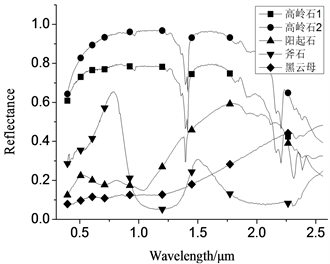Figure 2. Endmember spectral curves of five minerals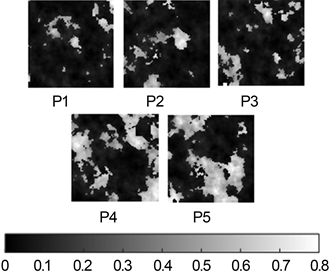Figure 3. Abundance of five mineral endmember

4.3. 参数的选择

$\lambda =\frac{1}{\sqrt{L}}{\sum }_{l}\frac{\sqrt{N}-{‖{x}_{l}‖}_{1}-{‖{x}_{l}‖}_{2}}{\sqrt{N-1}}$ (20)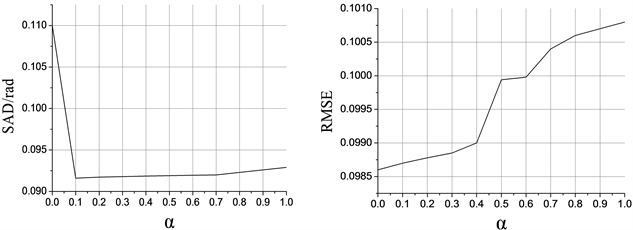Figure 4. Variation trend of SAD and RMSE with α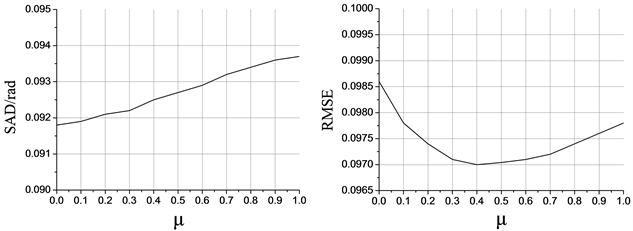Figure 5. Variation trend of SAD and RMSE with μ

4.4. 算法抗噪性检验Table 2. Results of kaolinite#1 and kaolinite#2 under different algorithms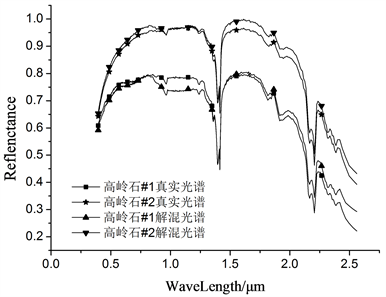Figure 6. Comparison of spectral curves of kaolinite#1 and kaolinite#2 obtained with real endmembers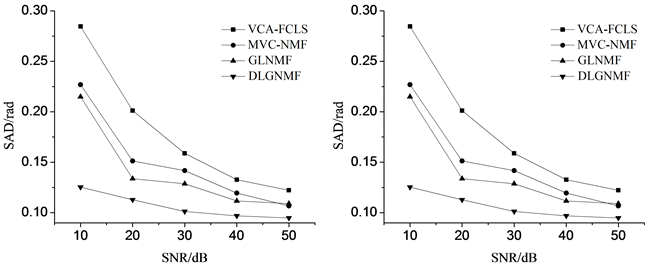Figure 7. SAD curves and RMSE curves of different algorithms under different SNR conditions

4.5. 图像大小对解混效果的影响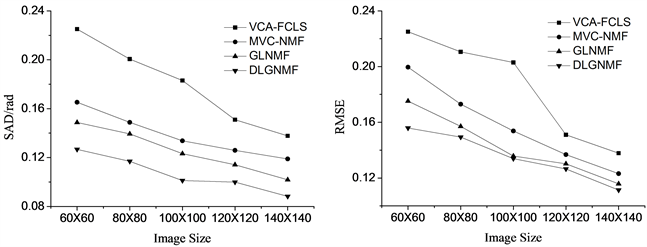Figure 8. SAD curves and RMSE curves of different algorithms under different image sizes

5. 真实高光谱数据实验验证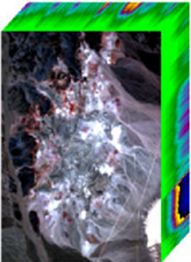Figure 9. AVIRIS hyperspectral data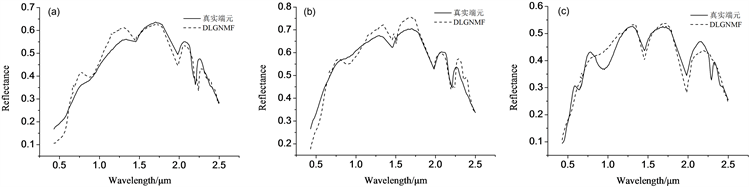Figure 10. Spectral curves corresponding to some endmembers. (a) Kaolinite#1; (b) Kaolinite#2; (c) Nontronite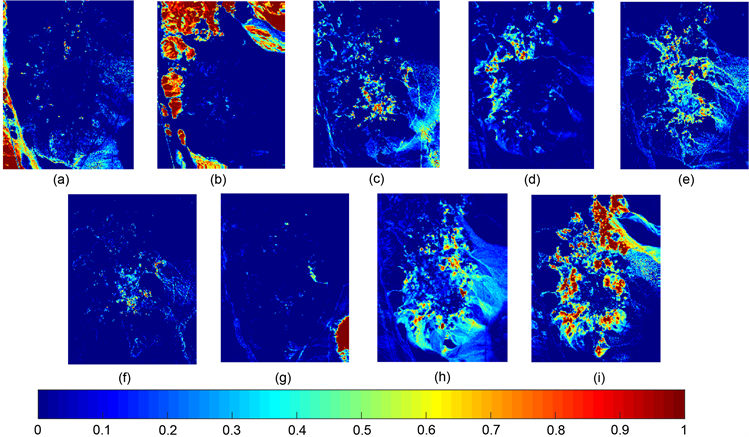Figure 11. Abundance corresponding to different endmembers. (a) Alunite; (b) Andradite; (c) Buddingtonite; (d) Dumortierite; (e) Kaolinite#1; (f) Kaolinite#2; (g) Montmorillonite; (h) Nontronite; (i) ChalcedonyTable 3. SAD and values obtained by different algorithms for this region

6. 结论

DLGNMF算法同时兼顾了图像的全局稀疏性与局部相似性，使得到的丰度矩阵更加符合真实端元分布。即使在处理噪声较大的高光谱数据时，解混精度更高，抗噪性能更好，结果更加稳定。

NOTES

*通讯作者。

 童庆禧, 张兵, 郑兰芬. 高光谱遥感——原理、技术与应用[M]. 北京: 高等教育出版社, 2006: 246-287.

 刘代志, 黄世奇, 王艺婷. 高光谱遥感图像处理与应用[M]. 北京: 科学出版社, 2016: 134-143.

 赵春晖, 王立国, 齐滨. 高光谱遥感图像处理方法及其应用[M]. 北京: 电子工业出版社, 2016: 66-76.

 张兵, 孙旭. 高光谱混合像元分解[M]. 北京: 科学出版社, 2015: 36-65.

 Shahed, S., Ahmad, R.M. and Moslem, F. (2019) The Use of Unmixing Technique in Stream Sediment Geochemical Exploration. Journal of Geochemical Exploration, 208, Article ID: 106339.
https://doi.org/10.1016/j.gexplo.2019.106339

 Lee, D.D. and Seung, H.S. (1999) Learning the Parts of Objects by Non-Negative Matrix Factorization. Nature, 401, 788-791.
https://doi.org/10.1038/44565

 赵岩. 高光谱图像线性模型混合像元分解的算法研究[D]: [硕士学位论文]. 哈尔滨: 哈尔滨理工大学, 2019.

 Jia, S. and Qian, Y.T. (2009) Constrained Nonnegative Matrix Factorization for Hyperspectral Unmixing. IEEE Transactions on Geoscience and Remote Sensing, 47, 161-173.
https://doi.org/10.1109/TGRS.2008.2002882

 Cai, D., He, X., Han, J., et al. (2011) Graph Regularized Nonnegative Matrix Factorization for Data Representation. IEEE Transactions on Pattern Analysis and Machine Intel-ligence, 33, 1548-1560.
https://doi.org/10.1109/TPAMI.2010.231

 Li, C., Gao, L., Plaza, A., et al. (2017) FPGA Implementation of a Maximum Volume Algorithm for Endmember Extraction from Hyperspectral Imagery. Workshop on Hyperspectral Image & Signal Processing: Evolution in Remote Sensing.

 Xu, J., Bobin, J., et al. (2019) Sparse Spectral Unmixing for Activity Estimation in γ-RAY Spectrometry Applied to Environmental Meas-urements. Applied Radiation and Isotopes.

 Wang, N., Du, B. and Zhang, L. (2013) An Endmember Dissimilarity Constrained Non-Negative Matrix Factorization Method for Hy-perspectral Unmixing. IEEE Journal of Selected Topics in Applied Earth Observations & Remote Sensing, 6, 554-569.
https://doi.org/10.1109/JSTARS.2013.2242255

 孙莉, 于瑞林, 吴杰芳. 非负矩阵分解与光谱解混[J]. 山东农业大学学报(自然科学版), 2019(5): 1-5.

 袁博. 基于混合像元空间与谱间相关性模型的NMF线性盲解混[J]. 测绘学报, 2019, 48(9): 1151-1160.

 唐茂峰. 高光谱图像高阶非线性混合像元分解算法研究[D]: [硕士学位论文]. 北京: 中国科学院大学(中国科学院遥感与数字地球研究所), 2018.

 Wang, X., Zhong, Y., Zhang, L., et al. (2017) Spatial Group Sparsity Regularized Nonnegative Matrix Factorization for Hyperspectral Unmixing. IEEE Transactions on Geoscience and Remote Sensing, 55, 6287-6304.
https://doi.org/10.1109/TGRS.2017.2724944

 Miao, L.D. and Qi, H.R. (2007) Endmember Extraction from Highly Mixed Data Using Minimum Volume Constrained Nonnegative Matrix Factorization. IEEE Transactions on Geoscience & Remote Sensing, 45, 765-777.
https://doi.org/10.1109/TGRS.2006.888466

 李登刚. 基于非负矩阵分解的高光谱图像混合像元分解方法研究[D]: [硕士学位论文]. 长沙: 湖南大学, 2016.

 Zhu, F.Y., Wang, Y., Fan, B., et al. (2014) Spectral Unmixing via Data-Guided Sparsity. IEEE Transactions on Image Processing, 23, 5412-5427.
https://doi.org/10.1109/TIP.2014.2363423

 刘雪松, 王斌, 张立明. 基于非负矩阵分解的髙光谱遥感凰像混合像元分解[J]. 红外与毫米波学报, 2011, 30(1): 27-32.

 Nascimento, J.M.P. and Dias, J.M.B. (2005) Vertex Component Analysis: A Fast Algorithm to Unmix Hyperspectral Data. IEEE Transactions on Geoscience and Remote Sensing, 43, 898-910.
https://doi.org/10.1109/TGRS.2005.844293

Top